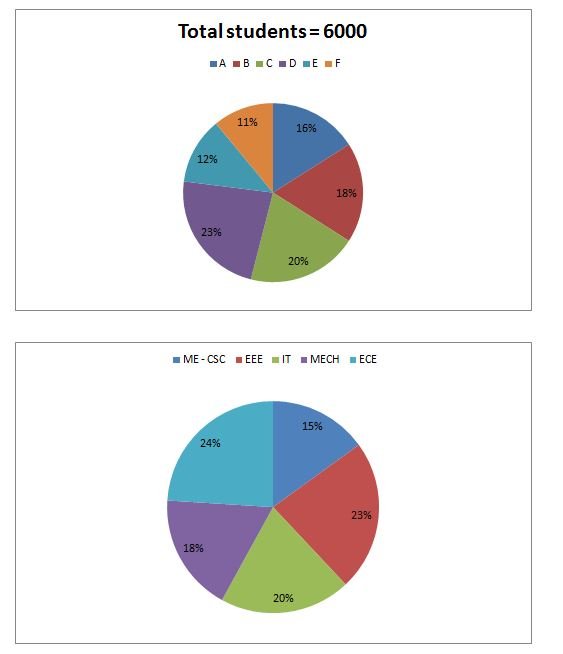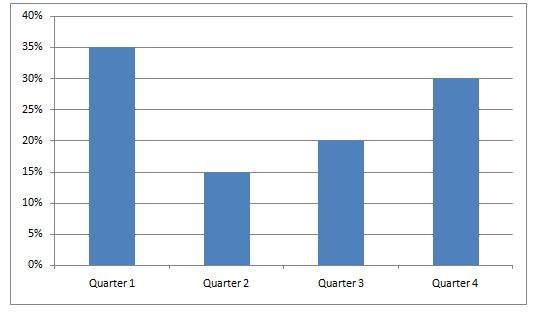# Quantitative Aptitude Questions (Data Interpretation) for IBPS RRB PO Prelims 2018 Day-164

Dear Readers, IBPS RRB is conducting Online Examination for the recruitment of probationary officer. To enrich your preparation here we have providing new series of Data Interpretation – Quantitative Aptitude Questions. Candidates those who are appearing in IBPS RRB PO Prelims Exams can practice these Quantitative Aptitude average questions daily and make your preparation effective.

Click “Start Quiz” to attend these Questions and view Solutions

Directions (1 – 5): Study the following information carefully and answer the given questions:

Following pie chart 1 shows the total number of students in 6 different colleges and pie chart 2 shows the percentage of students in different streams in different colleges.(Streams percentage is common for all the colleges)1) Find the ratio between the total number of IT students in college E to that of total number of ME – CSC students in College A?

a) 12: 19

b) 4: 7

c) 21: 29

d) 1: 1

e) None of these

2) Find the difference between the total number of MECH students in college B and E together to that of total number of ECE students in college C?

a) 36

b) 45

c) 54

d) 72

e) 18

3) If the ratio of male and female students in college F is 3 : 2 and the ratio of male and female IT students in the college C is 3 : 1, then find the sum of total number of female students in college F and the total number of male IT students in the college C?

a) 356

b) 228

c) 444

d) 390

e) None of these

4) Total number of students in college E is approximately what percentage of total number of ME-CSE students in college B?

a) 320 %

b) 444 %

c) 370 %

d) 480 %

e) 250 %

5) Find the average number of ME-CSC, EEE and MECH students in college C?

a) 224

b) 428

c) 316

d) 405

e) None of these

Directions (6 – 10): Study the following information carefully and answer the given questions:

Following table shows the total number of population in 5 different cities and the percentage of male among them.

 City Total population (In lakhs) % of male population A 8 42 % B 12 55 % C 15 60 % D 6 48 % E 10 53 %

The bar graph shows the percentage of males born in different quarters of month in different years. (Using total male population in all the given cities together)Note:

Quarter 1 starts from January to April.

6) Find the ratio between the total number of female population in city B to that of total number of male population in city D?

a) 12: 5

b) 22: 11

c) 13: 7

d) 15: 8

e) None of these

7) Find the difference between the total number of males born in quarter 1 to that of total number of female population in city E?

a) 479900

b) 425600

c) 385900

d) 327800

e) None of these

8) Find the total number of males born in Quarter 2, 3 and 4 together?

a) 1564200

b) 1687500

c) 1764100

d) 1925700

e) None of these

9) Total number of population in city A and B together is approximately what percentage of total number of female population in city C, D and E together?

a) 162 %

b) 175 %

c) 130 %

d) 145 %

e) 100 %

10) Total number of male population in city C is approximately what percentage more/less than the total number of female population in city A?

a) 115 % less

b) 95 % more

c) 80 % more

d) 65 % less

e) 125 % more

Direction (1-5):

The total number of IT students in college E

= > 6000*(12/100)*(20/100) = 144

The total number of ME – CSC students in College A

= > 6000*(16/100)*(15/100) = 144

Required ratio = 144: 144 = 1: 1

The total number of MECH students in college B and E together

= > 6000*[(18 + 12)/100]*(18/100) = 324

The total number of ECE students in college C

= > 6000*[(20)/100]*(24/100) = 288

Required difference = 324 – 288 = 36

The ratio of male and female students in college F = 3: 2

Total number of female students in college F

= > 6000*(11/100)*(2/5) = 264

The ratio of male and female IT students in the college C = 3: 1

Total number of male IT students in the college C

= > 6000*(20/100)*(20/100)*(3/4) = 180

Required sum = 264 + 180 = 444

Total number of students in college E = 6000*(12/100) = 720

Total number of ME-CSE students in college B

= > 6000*(18/100)*(15/100) = 162

Required % = (720/162)*100 = 444 %

The total number of ME-CSC, EEE and MECH students in college C

= > 6000*(20/100)*(15 + 23 + 18)/100 = 672

Required average = 672/3 = 224

Direction (6-10):

The total number of female population in city B

= > 12*(45/100)

The total number of male population in city D

= > 6*(48/100)

Required ratio = [12*(45/100)]: [6*(48/100)] = 15: 8

The total number of males born in quarter 1

= > [8*(42/100) + 12*(55/100) + 15*(60/100) + (6*48/100) + 10*(53/100)]*(35/100)

= > [3.36 + 6.6 + 9 + 2.88 + 5.3]*(7/20) = 9.499 lakhs

The total number of female population in city E

= > 10*(47/100) = 4.7 lakhs

Required difference = 9.499 – 4.7 = 4.799 lakhs = 479900

The total number of males born in Quarter 2, 3 and 4 together

= > 27.14*(65/100) = 17.641 lakhs = 1764100

Total number of population in city A and B together

= > 8 + 12 = 20 lakhs

Total number of female population in city C, D and E together

= > 15*(40/100) + 6*(52/100) + 10*(47/100)

= > 6 + 3.12 + 4.7 = 13.82 lakhs

Required % = (20/13.82)*100 = 145 %

Total number of male population in city C

= > 15*(60/100) = 9 lakhs

Total number of female population in city A

= > 8*(58/100) = 4.64 lakhs

Required % = [(9 – 4.64)/4.64]*100 = 94 % = 95 % more

Daily Practice Test Schedule | Good Luck

 Topic Daily Publishing Time Daily News Papers & Editorials 8.00 AM Current Affairs Quiz 9.00 AM Quantitative Aptitude “20-20” 11.00 AM Vocabulary (Based on The Hindu) 12.00 PM General Awareness “20-20” 1.00 PM English Language “20-20” 2.00 PM Reasoning Puzzles & Seating 4.00 PM Daily Current Affairs Updates 5.00 PM Data Interpretation / Application Sums (Topic Wise) 6.00 PM Reasoning Ability “20-20” 7.00 PM English Language (New Pattern Questions) 8.00 PM